﻿ cru
Cube Root Unity Complex Fuctions

Author: Renzo Diomedi

e_mail : rnzxyz@outlook.it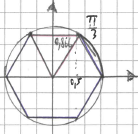Well known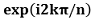with k and n natural numbers, is a root of unity with value = 1 and if n=3 is a cube root of unity , a complex number that creates an equilateral Triangle (since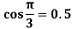), hence a Hexagon. So well known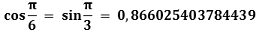,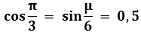Unlike the hexagonal lattice created by the Eisenstein number, the points (or vectors) of this Lattice are not all algebraic entities.

It has 3 orthogonal axes: x , y , z and 6 non-orthogonal axes which are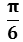oblique :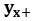,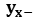,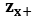,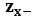,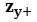,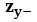if x = y = z they are in a Sphere that contains 3 crisscross regular hexagones

then the coordinates liying on these 9 space-dimensions are 8 : 0,5x ; -0,5x ; 0,866025y ; -0,866025y ; 0,5y ; -0,5y ; 0,866025z ; -0,866025z and their resulting points are 16 that, joining the external points, create a cuboid volume

If x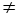yz the coordinates liying on these 9 space-dimensions , considering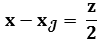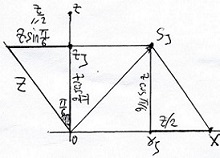are 10 : x-0,5y ; -x+0,5y ; x-0,5z ; -x+0,5z ; 0,866025y ; -0,866025y ; y-0,5z ; -y+0,5z ; 0,866025z ; -0,866025z and their resulting points are 32 that, joining the external points, create a 14-sided polyhedron that contains 2 orthogonally crossed irregular identical hexagones around the Z axis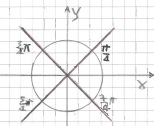the Output of this function is a closed and compact space around the point of origin of the axes. It is a large point that replaces the ordinary single minimal point. This volume is modified by the Time, that here is the 10th Dimension, using a D'Alembert operator extended on all 9 axes, thus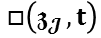where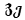is a 3D conjugate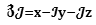with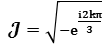. Vectors move form every of 16 or 32 points in directions of its new position. Time to time from older to next positionof the points and so on.
the spacetime deformation of the 14-sided Polyhedron measures...........

..............................to be continued